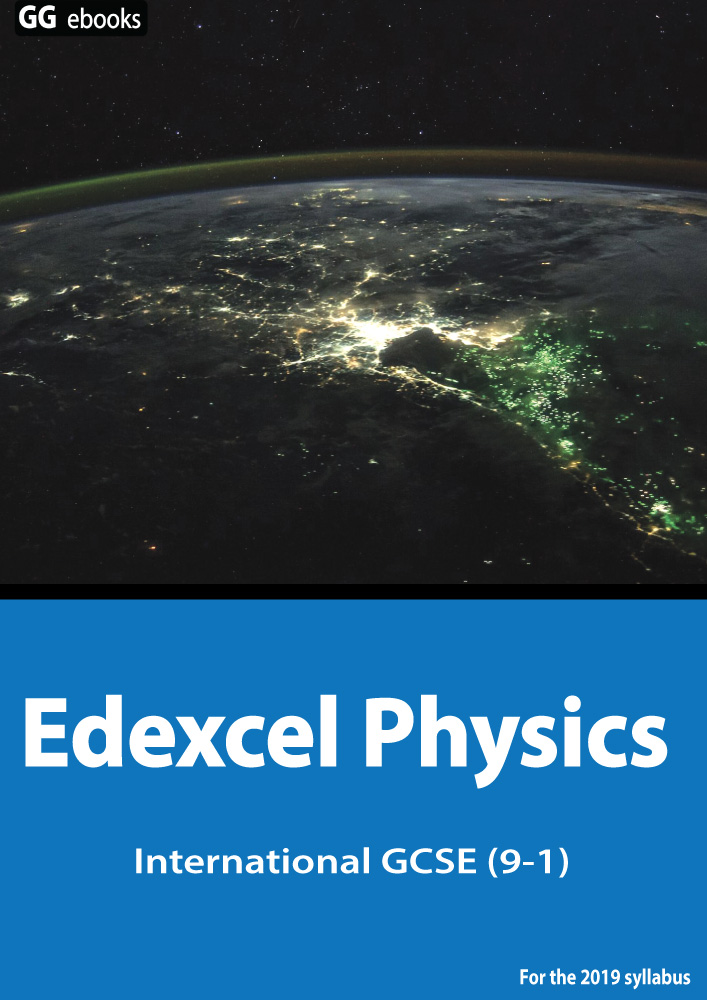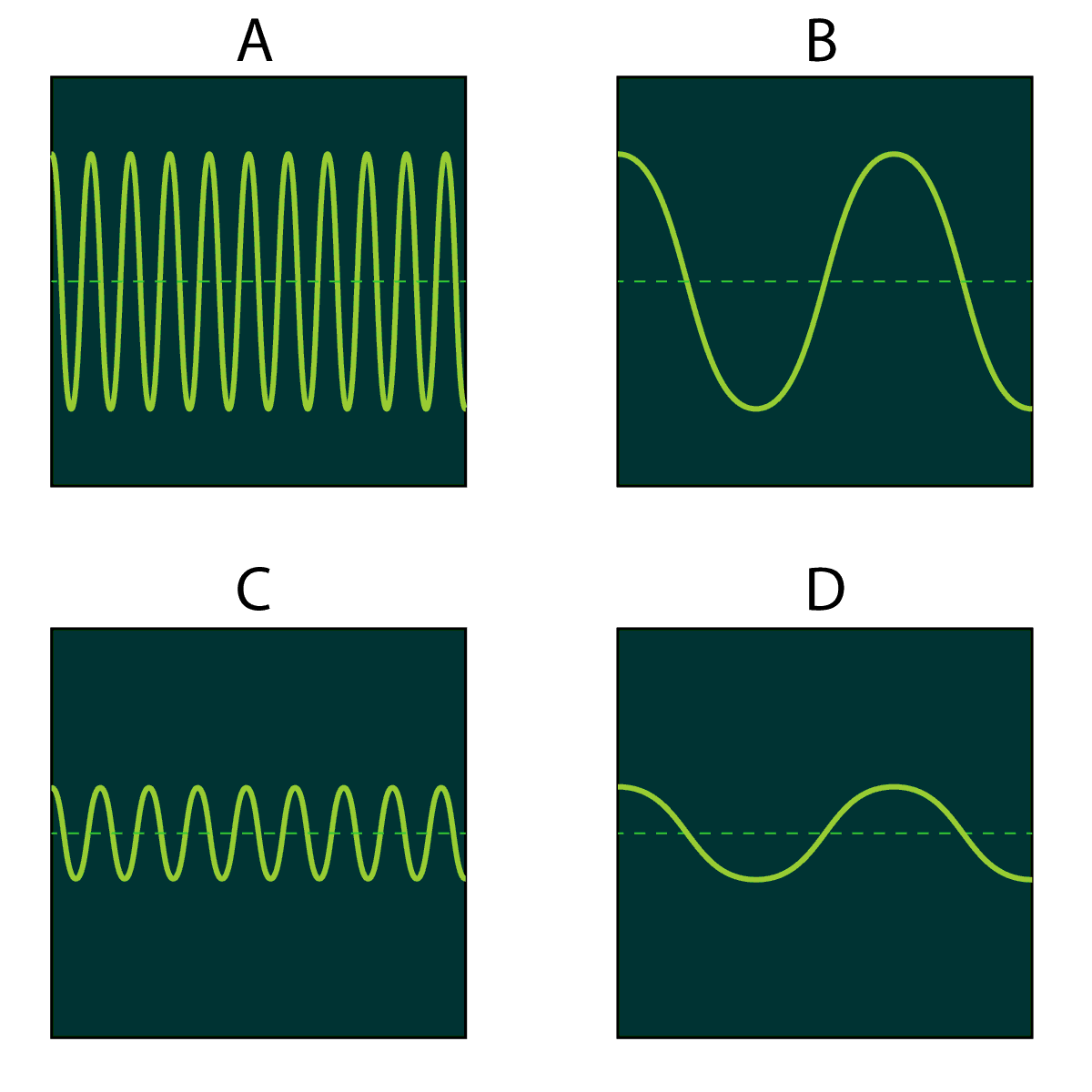Edexcel iGCSE - Triple Extras

Page 1

 NEED HELP? Edexcel iGCSE digital textbooks From the makers of GradeGorilla. GGebooks.com (Opens in a new tab)Sound, Forces, Energy Resources, Magnetism, Transformers. 1. What is the highest frequency humans can hear? A). 10 kHz B). 20 kHz C). 100 kHz D). 200 kHz ? A B C D 2. What is the lowest frequency humans can hear? A). 10 Hz B). 20 Hz C). 100 Hz D). 200 Hz ? A B C D 3. What do you call a device with a screen that can be used with a microphone to display a sound wave? A). Oscilloscope B). Stethoscope C). Sound sensor D). Signal generator ? A B C D 4&5. Here are 4 sound waves shown on one of these devices.Which of these diagrams shows... 4. A loud, high pitched sound? ? A B C D 5. A quiet high frequency sound? ? A B C D 6. A low frequency sound with a high amplitude? ? A B C D 7&8. In an experiment to measure the speed of sound, a student bangs together 2 wooden blocks. Another student listens out for the echo from the wall. The wall is 100 m away. The echo returns to the students after a delay of 0.6 seconds.7. Which of these 4 formulas should be used to calculate the speed of sound? A). speed = velocity x time B). speed = distance x time C). speed = velocity ÷ time D). speed = distance ÷ time ? A B C D 8. Using the data from this experiment, what is the speed of sound? A). 60 m/s B). 167 m/s C). 120 m/s D). 333 m/s ? A B C D 9. Which of the points on this metal block shows the position of the centre of gravity?? A B C D 10. The diagram below shows a light wooden beam supported at each end. A 1000 N weight rests on the beam closer to the right hand side of the beam.Which of the answers below gives realistic values for the size of the forces X and Y? A). X = 700 N, Y = 300 N. B). X = 300 N, Y = 700 N. C). X = 500 N, Y = 500 N. D). X = 1000 N, Y = 1000 N. ? A B C D